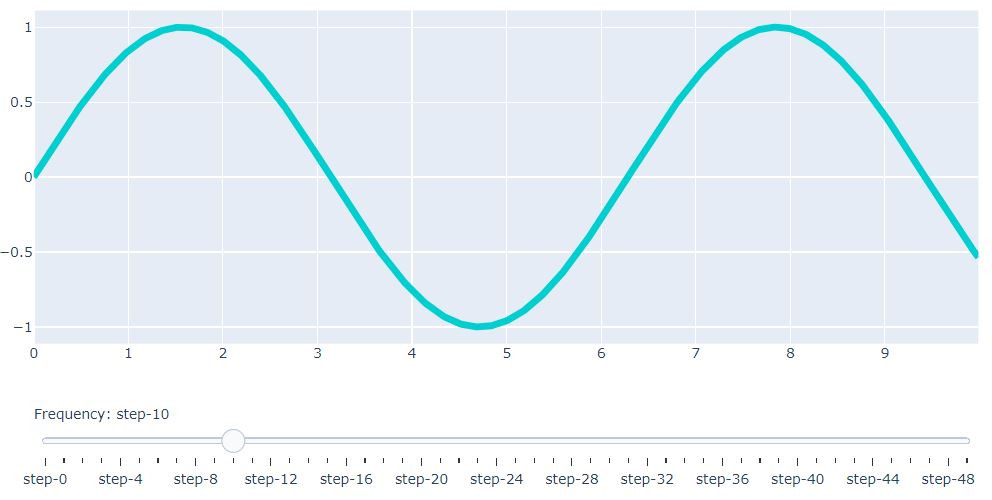DAY 11
0
AI & Data

Day11 Data Visualization Tools: Plotly 視覺化資料工具：Plotly

Plotly是使用Python和Django框架構建的，前端使用JavaScript和可視化庫D3.js，HTML和CSS。使用Plotly可以創建出許多精美的互動式圖表。
Plotly was built using Python and the Django framework, with a front end using JavaScript and the visualization library D3.js, HTML and CSS. Plotly is a graphing library for making interactive graphs.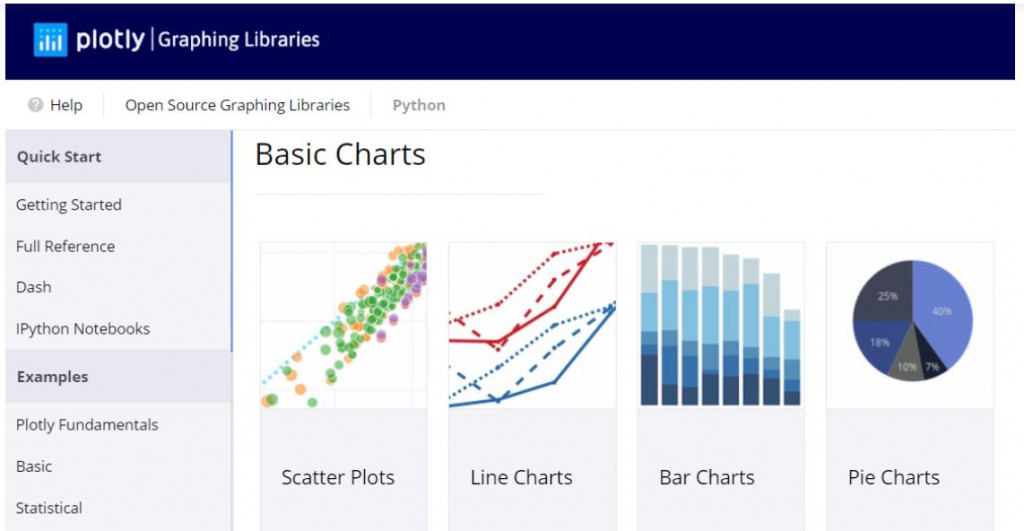將載入的資料繪製成表格與基礎圖表

Import Data (in this example is a csv hosted on github) and display it in a table and a basic plot using Plotly.

from plotly.offline import iplot
import plotly.figure_factory as ff
import pandas as pd

table = ff.create_table(df)
iplot(table, filename='jupyter-table1')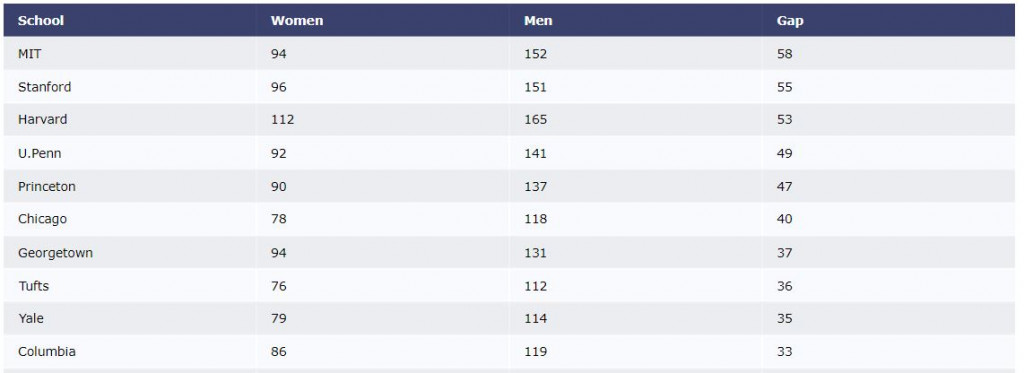import plotly.graph_objects as go

schools = df.School
data = [go.Bar(x=df.School,
y=df.Gap)]

iplot(data, filename='jupyter-basic_bar')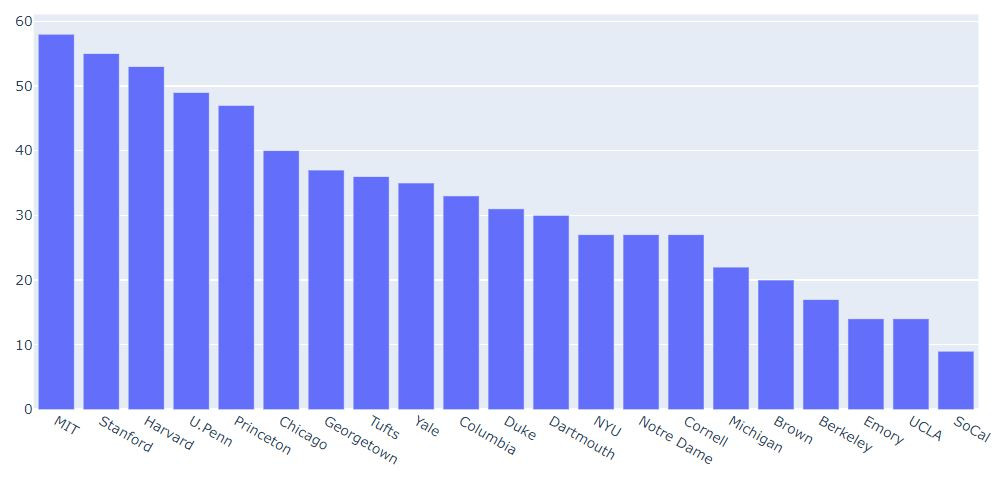繪製3D地表地形圖 Topographical 3D Surface Plot

We can make sophisticated interactive 3D plots using Numpy and Plotly.

import plotly.graph_objects as go
import pandas as pd

# Read data from a csv

fig = go.Figure(data=[go.Surface(z=z_data.values)])

fig.update_layout(title='Mt Bruno Elevation', autosize=False,
width=500, height=500,
margin=dict(l=65, r=50, b=65, t=90))

fig.show()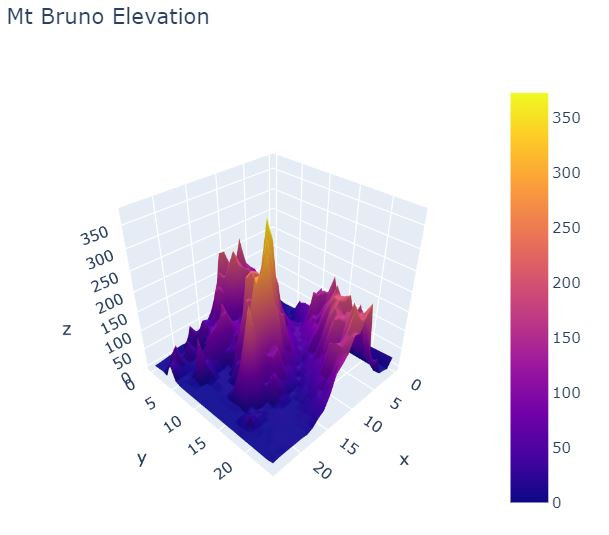功能按鈕 Plot Controls & IPython widgets

We can also add plot controls and IPython widgets through Plotly, such as sliders or buttons.

import numpy as np

data = [dict(
visible = False,
line=dict(color='#00CED1', width=6),
name = '? = '+str(step),
x = np.arange(0,10,0.01),
y = np.sin(step*np.arange(0,10,0.01))) for step in np.arange(0,5,0.1)]
data['visible'] = True

steps = []
for i in range(len(data)):
step = dict(
method = 'restyle',
args = ['visible', [False] * len(data)],
)
step['args'][i] = True # Toggle i'th trace to "visible"
steps.append(step)

sliders = [dict(
active = 10,
currentvalue = {"prefix": "Frequency: "},
steps = steps
)]

layout = dict(sliders=sliders)
fig = dict(data=data, layout=layout)

iplot(fig, filename='Sine Wave Slider')